﻿ 5. Detailed description of the Actions > 5.5. Standard > 5.5.2. Sort (High-Speed action) > 5.5.2.6. Managing RAM memory 3.

# 5.5.2.6. Managing RAM memory 3.

Let’s consider the following data transformation graph: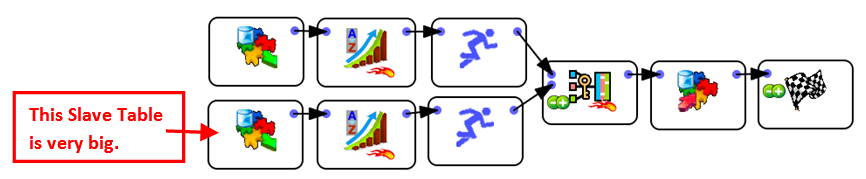This is a very common situation: We need to join the two tables using the simple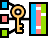Join Action (because the Slave Table is very big and we can’t use the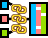MultiJoin Action with very big Slave Table). To use the simpleJoin Action, we first need to sort the two tables. To (roughly) divide the computation time by two, we’ll sort the two tables in parallel (i.e. at the same time), using two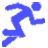Multithread Actions. Indeed, in the above graph, 95% of the computation time is used to compute the 2 sorts (sorting is nearly always the slowest Action that you can do inside any ETL).

Let’s assume that, for each of the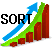Sort Action, the “Memory Buffer Size” parameter is 1200MB. This means that the total amount of RAM memory used by Anatella to execute this graph is 2x1200MB=2400MB=2.4GB (i.e. this graph won’t run on a 32-bit computer)(because the twoSort Actions are running simultaneously and their respective memory consumption adds up).

To be able to run this graph on a 32-bit computer (i.e. using less than 2GB RAM), you can either:

Decrease the “Memory Buffer Size” parameter of the twoSort Actions to 800MB.

Remove the twoMultithread Actions. The whole graph will then execute sequentially. More precisely, we’ll have:

1.Anatella creates all the tape files to sort the first table. To compute the tape files, Anatella requires 1200MB (i.e. the “Memory Buffer Size” is 1200MB). The amount of free RAM memory is 2GB-1200MB=800MB.

2.Let’s assume that there are 30 tape files and that they are now all computed. Anatella free’s the RAM memory that was required to compute the tape files and opens all the tape files to fusion them (using the Merge Sort algorithm). The amount of free RAM memory is now 2GB-30x10MB=1700MB (i.e. we lost 300MB to open the 30 tape files).

3.Anatella creates all the tape files to sort the second table. To compute the tape files, Anatella requires 1200MB. The amount of free RAM memory is 1700MB-1200MB=500MB.

4.Let’s assume that there are 25 tape files and that they are now all computed. Anatella free’s the RAM memory that was required to compute the tape files and opens all the tape files to fusion them (using the Merge Sort algorithm). The amount of free RAM memory is now 1700MB-25x10MB=1450MB (i.e. we lost 250MB to open the 25 tape files).

5.The twoSort Actions are using the 30+25=55 tape files to produce, row-by-row, the two sorted table (All these 55 tape files are opened simultaneously). The 2 sorted tables are used, row-by-row, to compute the simpleJoin Action. The amount of free RAM memory during the join is 1450MB (i.e. we lost 550MB out of 2GB to open the 55 tape files).